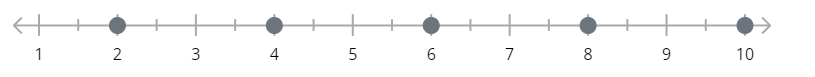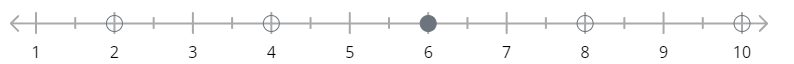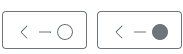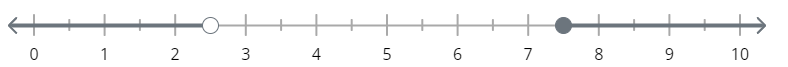### How Do I Use the Number line Tool?

The Number Line tool lets you define a number line and allow your students to answer questions by plotting points, selecting pre-plotted points, or defining a range of possible values. To create a Number Line item, follow these instructions.

## Insert a Number Line Item

Bring up the Insert popup by clicking or drawing a rectangle anywhere on your activity. Then choose "Number Line."## Set the Score Points

In the Number Line dialog, set "Score Points" to the point value a student will be awarded for answering correctly.

## Set Up Your Number Line

The default settings draw the line from 0 to 10 in whole number steps. You can change the display by following these instructions:

• Change the end points by setting the Start and Stop values.
• Click Advanced Options to set decimal intervals and choose how often the labels are drawn
• Enter the "Step" value to indicate how often a tick mark will be drawn on the line. Enter a decimal value to drawn tickmarks between whole numbers. For example, entering .5 draws tickmarks at points 0, 0.5, 1.0, 1.5, etc.
• Set "Marks per Label" to indicate how often a label should be drawn. For instance, setting it to "2" indicates a label will be drawn every other tick mark.
• You can hide or show the start label and end label. This is useful if you want to create some padding on the number line around the endpoints.

Here's an example with tick marks every 0.5, labels drawn every other tick mark, and the Start label turned off:Note that the Number Line limits the tick marks to a maximum of 50. You will see a message if your set up exceeds that maximum.

## Choose the Interaction Type

The Number Line item lets you choose between three interactions. The prompt for the question should be provided either in the activity background that you uploaded or in a Teacher Text box that you add near the Number Line interaction.

 Plot Points Students will click on one or more tick marks to indicate which point(s) answer the question. Select Points Some points will already be drawn on the number line and the student will choose which of them answers the question.This is a good choice to focus the student's decision making. If there are a lot of points to choose from, you may be better off choosing "Plot Points." Plot Intervals Student will indicate the endpoint of a ray, and whether their answer is inclusive of the endpoint. This is a good choice for mapping inequalities on a number line.

To set up the answer key for each item type, you will click on points on the number line. The specific process for each interaction is defined below.

## How To Set Up a "Plot Points" Question

Click on every tick mark that must be plotted by the student to answer correctly. For example, this is the answer key for a question prompting students to "Click each even whole number."The student must click all of the indicated tick marks to receive the Score Points.

How To Set Up a "Select Points" Question

The Select Points question plots some points on the number line and the student must choose one or more of those drawn points to indicate their answer.

First, click tick marks to choose which points are to be drawn. Then drawn points to indicate which should be chosen by students to be scored as correct.

For example, this is the answer key for a question prompting students to indicate "Which of these even numbers are also divisible by 3?"Students would be able to select any or all of the drawn points. If you want them to choose only one point, turn on the "Limit Number of Selections" option.The "Limit Number of Selections" option means that the students will not be allowed to select more points than are indicated correct in the answer key. In the above example, students would only be able to select one point since there is a single correct answer. Subsequent clicks on other points would deselect their answer and choose a new point.

## How To Set Up a "Plot Intervals" Question

This question type is intended to let students map inequalities on the number line by plotting up to two rays - one pointing left, the other pointing right.

Add an interval to the number line g line by clicking one of these options for left-pointing rays (i.e. "less than" inequalities):or one of these for right-pointing (i.e. "greater than") rays:The solid point indicates that the interval includes the endpoint and the hollow point indicates that the inequality excludes that endpoint.

Drag the endpoint of the newly drawn interval to the correct tick mark.

Repeat if required to add the opposite facing interval.

This example allows students to map the inequality "x < 2.5 or x >= 7.5"To remove either interval from your answer key, click the appropriate "Remove" button.

Students answer these questions by choosing left- and right-pointing rays, then dragging the endpoint to the correct tickmark.

## Scoring

Students must completely answer the question to receive the score points, and partial credit is not awarded. For instance, if you ask the student to plot 1, 2, and 3 on the number line, they must plot all three points correctly to receive the Score Points set for the question.